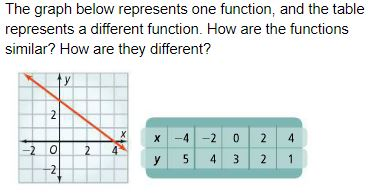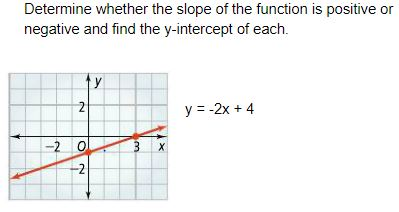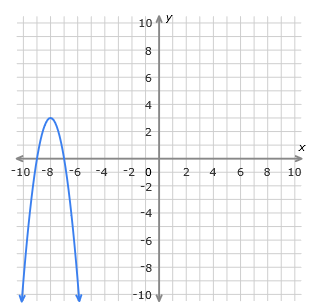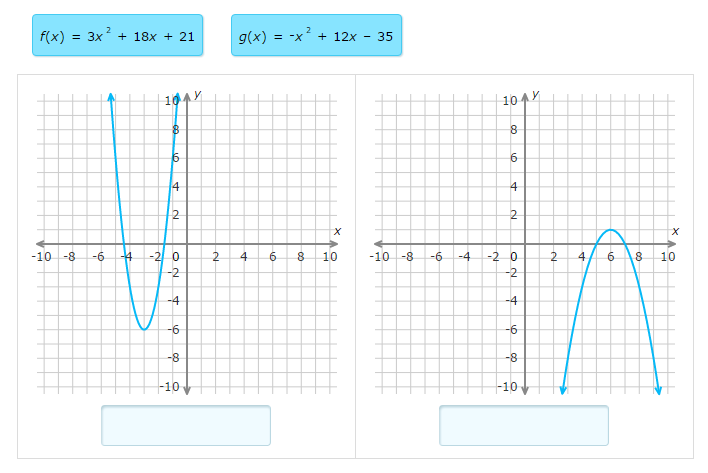#### MAT-HS.F-IF.09

 MAT-HS Targeted Standards(F) Concept: Function(IF) Domain: Interpreting FunctionsCluster: Analyze functions using different representations. MAT-HS.F-IF.09* Compare properties of two functions each represented in a different way (algebraically, graphically, numerically in tables, or by verbal descriptions).

## Student Learning Targets:

• I can
• I can

• I can

### Skills (Performance) Targets

• I can compare the key features of two functions that are represented in different ways.

• I can
• I can

## Proficiency Scale

 Score Description Sample Activity 4.0 (advanced) In addition to achieving level 3.0 content, the student makes in-depth inferences and applications that go beyond what was taught Given the function, y = 3x + 2 create the following: A graph that is increasing at the same rate. A table that is increasing at a lesser rate A verbal description that is increasing at a greater rate. Graph 2x + 3y = 6, 2x + 3y = 12, and 2x + 3y = 18 in the same coordinate plane. . How are the lines from part (a) related? . As C increases, what happens to the graph of 2x + 3y = C 3.5 In addition to Score 3.0 performance, the student demonstrates in-depth inferences and applications regarding the more complex content with partial success. 3.0 (proficient) The student can: compare properties of two functions each represented in a different way: algebraically graphically numerically in tables verbal descriptions The student exhibits no major errors or omissions.The ordered pairs below represent one function, and the verbal description represents a different function. How are the functions similar? How are they different?   (0, 2), (1, 4), (2, 6), (3, 8)   This function has a slope of - ½ and a y-intercept of -32.5 The student demonstrates no major errors or omissions regarding the simpler details and processes (Score 2.0 content) and partial knowledge of the more complex ideas and processes (Score 3.0 content). 2.0 (progressing) There are no major errors or omissions regarding the simpler details and processes as the student: can recognize and recall terminology such as: equation, maximum, minimum, x and y intercept, rate of change, slope can identify properties of a function, but can not compare with another function However, the student exhibits major errors or omissions regarding the more complex ideas and processes.1.5 The student demonstrates partial knowledge of the simpler details and processes (Score 2.0 content) but exhibits major errors or omissions regarding the more complex ideas and procedures (Score 3.0 content). 1.0 (beginning) With help, a partial understanding of some of the simpler details and processes and some of the more complex ideas and processes. - 0.5 With help, the student demonstrates a partial understanding of some of the simpler details and processes (Score 2.0 content) but not the more complex ideas and processes (Score 3.0 content).

## Alg II Comparing Functions Proficiency Scale

Score   Description Sample Activity

4.0

In addition to Score 3.0, the student demonstrates in-depth inferences and applications regarding more complex material that go beyond end of instruction expectations.

Which of the functions have a larger increasing interval on the domain from [-2, 3]?

f(x)=ex-3+2   g(x)=x3-4x

3.5 In addition to Score 3.0 performance, the student demonstrates in-depth inferences and applications regarding the more complex content with partial success.

3.0

(proficient)

The student can:
• compare the key features of two functions that are represented in different ways.

The student exhibits no major errors or omissions.

Determine which of the functions presented has the largest maximum value.

f(x)=2x2-28x+90

g(x)=(x+3)(x-2)

Compare the two functions below.  Determine the x-intercept they both share.x y -14 -2 -7 0 0 2 7 4
2.5 The student demonstrates no major errors or omissions regarding the simpler details and processes (Score 2.0 content) and partial knowledge of the more complex ideas and processes (Score 3.0 content).

2.0

(progressing)

There are no major errors or omissions regarding the simpler details and processes as the student can:
• classify a function given a table, graph, or equation.

• find the key features of a graph, a table, or an equation.
• recognize and recall basic terminology, such as:
• linear
• vertex
• exponential
• intercept
• maximum/minimum
• line of symmetry
• increasing/decreasing intervals
• opens up/opens down

However, the student exhibits major errors or omissions regarding the more complex ideas and processes.

Match the correct equation to the correct graph.Given the two equations, decide which function has the greater slope.     f(x)=2x+9
 x y -2 -3 0 -2 2 -1 4 0
1.5 The student demonstrates partial knowledge of the simpler details and processes (Score 2.0 content) but exhibits major errors or omissions regarding the more complex ideas and procedures (Score 3.0 content).

1.0

(beginning)

With help, the student demonstrates a partial understanding of some of the simpler details and processes (Score 2.0 content) and some of the more complex ideas and processes (Score 3.0 content).
0.5 With help, the student demonstrates a partial understanding of some of the simpler details and processes (Score 2.0 content) but not the more complex ideas and processes (Score 3.0 content).
0.0 Even with help, the student demonstrates no understanding or skill.

## Resources

 Web Vocab